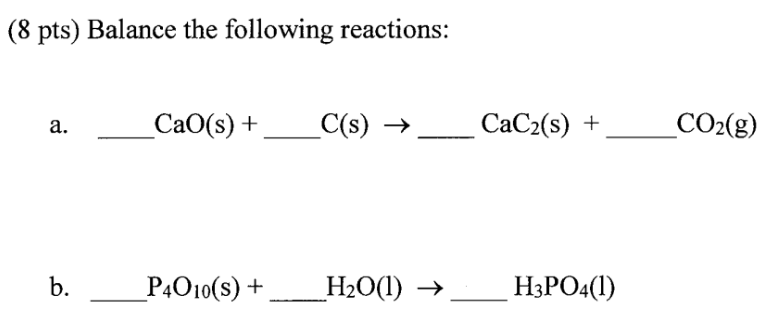# Problem: Balance the following reactions: a. _____CaO (s) + _____C (s) → _____CaC 2 (s) + _____CO2 (g) b. _____P4O10 (s) + _____H2O (l) → _____H3PO4 (l)

###### FREE Expert Solution
87% (456 ratings)###### Problem Details

Balance the following reactions:

a. _____CaO (s) + _____C (s) → _____CaC (s) + _____CO2 (g)

b. _____P4O10 (s) + _____H2O (l) → _____H3PO4 (l)